# 7.2 The General Quadratic Equation

The analytic equation for a conic in arbitrary position is the following: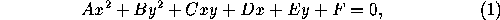where at least one of A, B, C is nonzero. To reduce this to one of the forms given previously, perform the following steps (note that the decisions are based on the most recent values of the coefficients, taken after all the transformations so far):

1.   If C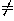0, simultaneously perform the substitutions x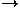qx+y and yqy-x, where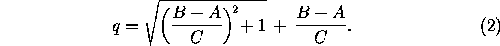Now C=0. (This step corresponds to rotating and scaling about the origin.)

2.   If B=0, interchange x and y. Now B0.
3.   If E0, perform the substitution yy-½(E/B). (This corresponds to translating in the y direction.) Now E=0.
4. If A=0:
1.   If D0, perform the substitution xx-(F/D) (translation in the x direction) and divide the equation by B to get (3) . The conic is a parabola.
2. If D=0, the equation gives a degenerate conic. If F=0, we have the line y=0 with multiplicity two. If F<0 we have two parallel lines y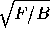. If F>0 we have two imaginary lines; the equation has no solution over the real numbers.
5. If A0:
1. If D0, perform the substitution xx-½(D/A). Now D=0. (This corresponds to translating in the x direction.)
2. If F0, divide the equation by F to get a form with F=1.
1. If A and B have opposite signs, the conic is a hyperbola; to get to (2) , interchange x and y if necessary so that A is positive, then make a=1/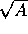and b=1/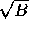.
2. If A and B are both positive, the conic is an ellipse; to get to (1) , interchange x and y if necessary so that AB, then make a=1/and b=1/. The circle is the particular case a=b.
3. If A and B are both negative, we have an imaginary ellipse; the equation has no solution over the real numbers.
3. If F=0, the equation again represents a degenerate conic: when A and B have different signs we have a pair of lines yx, and when they have the same sign we get a point (the origin).

We work out an example for clarity. Suppose the original equation is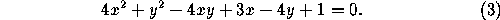In step 1 we apply the substitutions x2x+y and y2y-x. This gives 25x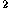+10x-5y+1=0. Next we interchange x and y (step 2) and get 25y+10y-5x+1=0. Replacing y by y-in step 3 we get 25y-5x=0. Finally, in step 4a we divide the equation by 25, thus giving it the form (3) with a=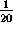. We have reduced the conic to a parabola with vertex at the origin and focus at (,0). To locate the features of the original curve, we work our way back along the chain of substitutions (recall the convention about substitutions and transformations from Section 1.1):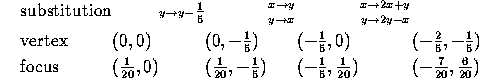We conclude that the original curve (3) is a parabola with vertex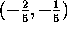and focus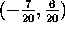.

If one just wants to know the type of the conic defined by (1) , an alternative analysis consists in forming the quantities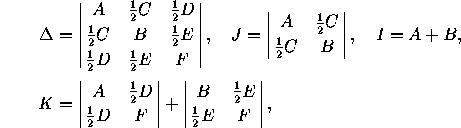and finding the appropriate case in the following table, where an entry in parentheses indicates that the equation has no solution over the real numbers: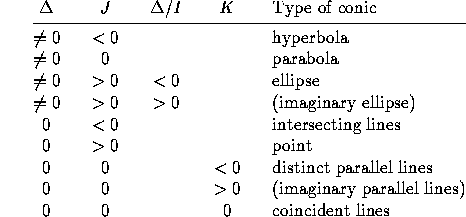For the central conics (the ellipse, the point, and the hyperbola), the center (x,y) is the solution of the system of equations

2Ax+Cy+D=0,
Cx+2By+E=0,

and the axes have slope q and -1/q, where q is given by (2) .

Next: 7.3 Additional Properties of Ellipses
Up: 7 Conics
Previous: 7.1 Alternative CharacterizationThe Geometry Center Home Page

Silvio Levy
Wed Oct 4 16:41:25 PDT 1995

This document is excerpted from the 30th Edition of the CRC Standard Mathematical Tables and Formulas (CRC Press). Unauthorized duplication is forbidden.